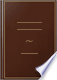Αναζήτηση Εικόνες Χάρτες Play YouTube Ειδήσεις Gmail Drive Περισσότερα »
Είσοδος
 Βιβλία Βιβλία 21 - 30 από 192 για All the interior angles of any rectilineal figure, together with four right angles,....All the interior angles of any rectilineal figure, together with four right angles, are equal to twice as many right angles as the figure has sides.Elements of Plane Geometry: For the Use of Schools - Σελίδα 83
των Nicholas Tillinghast - 1844 - 96 σελίδες
Πλήρης προβολή - Σχετικά με αυτό το βιβλίο## Elements of arithmetic, algebra and geometry

George Lees - 1826 - 207 σελίδες
...all the angles of the figure, together with four right angles ; that is, the angles of the figure are equal to twice as many right angles, as the figure has sides wanting four. PROP. XIII. THEOREM. If two triangles, BAG, EOF, have two angles, BAG, ABC, and a side...## Elements of Geometry: With Notes

John Radford Young - 1827 - 208 σελίδες
...in each triangle amounts to two right angles, therefore the angles of all the triangles are together equal to twice as many right angles as the figure has sides, that is to say, the sum of the angles of the polygon, together with those about the point within it,...## The Elements of Euclid: The Errors by which Theon, Or Others, Have Long ...

Robert Simson - 1827 - 513 σελίδες
...zi. COR. 1. All the interior angles of any rectilineal figure, together with four right angles, are equal to twice as many right angles as the figure has sides. For any rectilineal figure ABCDE, can be divided into as many triangles as the figure has sides, by...## The First Six Books of the Elements of Euclid, with a Commentary and ...

Euclid, Dionysius Lardner - 1828 - 324 σελίδες
...Hence it follows, that the sum of all the angles internal and external, including the reentrant angles, is equal to twice as many right angles as the figure has sides, together with the excess of every reentrant angle above two right angles. But (134) the sum of the...## Elements of the Geometry of Planes and Solids: With Four Plates

Ferdinand Rudolph Hassler - 1828 - 159 σελίδες
...angles, as AGD, GDE, and so on, standing in equal segments, are equal to one another; and their sum being equal to twice as many right angles as the figure has sides wanting four: that is, eight right angles, each of these angles of the hexagon is equal eight sixths...## Elements of geometry: Being chiefly a selection from Playfair's geometry

John Playfair - 1829 - 186 σελίδες
...diagonals &c. QED PROPOSITION L. THEOREM. All the interior angles of any rectilineal figure arc together equal to twice as many right angles as the figure has sides, wanting four right angles'. Let ABCDE be any rectilineal figure; all its interior angles A, B, C, D,...## The London Encyclopaedia: Or, Universal Dictionary of Science, Art ..., Τόμος 10

Thomas Curtis - 1829
...118 119 липу right angles as the figure has sides. Hence the interior angles of the figure are equal to twice as many right angles as the figure has sides wanting four right angles. Cor. 1. All the interior angles of a quadrilateral figure are together equal...## Geometry, Plane, Solid, and Spherical, in Six Books: To which is Added, in ...

Pierce Morton - 1830 - 272 σελίδες
...together equal to four right angles ; and the sum of its interior angles, together with four right angles, is equal to twice as many right angles as the figure has sides . . • 15 (¿•) The area of a rectilineal figure may be obtained by dividing it into triangles,...## The first six books of the Elements of Euclid: with notes

Euclid, Thomas Elrington - 1833 - 183 σελίδες
...angle EDN ; therefore the reentrant angle, with the sum of all the other internal and external angles, is equal to twice as many right angles as the figure has sides, and to its excess EDN above two right angles : but the internal angles, with four right angles, are...## Elements of Geometry with Notes

John Radford Young - 1833 - 216 σελίδες
...interior opposite angle CDE. A•B PROPOSITION XVII. THEOREM. In any polygon the sum of all the angles is equal to twice as many right angles as the figure has sides, all but four right angles. For if from the vertices of the several angles, lines be drawn to any point...